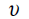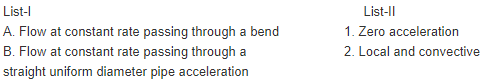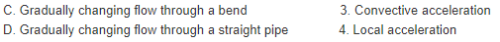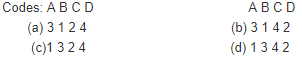Courses

# Hydrostatic Forces On Surfaces And Fluid Kinematics - MCQ Test 1

## 20 Questions MCQ Test Mock Test Series - Mechanical Engineering (ME) for GATE 2020 | Hydrostatic Forces On Surfaces And Fluid Kinematics - MCQ Test 1

Description
This mock test of Hydrostatic Forces On Surfaces And Fluid Kinematics - MCQ Test 1 for Mechanical Engineering helps you for every Mechanical Engineering entrance exam. This contains 20 Multiple Choice Questions for Mechanical Engineering Hydrostatic Forces On Surfaces And Fluid Kinematics - MCQ Test 1 (mcq) to study with solutions a complete question bank. The solved questions answers in this Hydrostatic Forces On Surfaces And Fluid Kinematics - MCQ Test 1 quiz give you a good mix of easy questions and tough questions. Mechanical Engineering students definitely take this Hydrostatic Forces On Surfaces And Fluid Kinematics - MCQ Test 1 exercise for a better result in the exam. You can find other Hydrostatic Forces On Surfaces And Fluid Kinematics - MCQ Test 1 extra questions, long questions & short questions for Mechanical Engineering on EduRev as well by searching above.
QUESTION: 1

Solution:

Ans. (c)

QUESTION: 2

Solution:

Ans. (a)

QUESTION: 3

### The 2-D flow with, velocity= (x + 2y + 2)i + (4 – y)j is:

Solution:

Ans. (d) Continuity equation satisfied but ω≠ 0

QUESTION: 4

Match List-I (Pipe flow) with List-II (Type of acceleration) and select the correct answer:Solution:

Ans. (a)

QUESTION: 5

Irrotational flow occurs when:

Solution:

Ans. (c) If the fluid particles do not rotate about their mass centres while moving in the direction of motion, the flow is called as an irrotational flow.

QUESTION: 6

Assertion (A): Stream lines are drawn in the flow field such thatat a given instant of time there perpendicular to the direction offlow at every point in the flow field.
Reason (R): Equation for a stream line in a two dimensional flowis given by Vx dy – Vy dx = 0.

Solution:

Ans. (d) A streamline in a fluid flow is a line tangent to which at any point is in the direction of velocity at that point at that instant.

QUESTION: 7

Which one of the following is the correct statement?
Streamline, path line and streak line are identical when the

Solution:

Ans (a)

QUESTION: 8

The continuity equation for a steady flow states that

Solution:

Ans. (c) It is a possible case of fluid flow therefore the stream function exists for steady flows.

QUESTION: 9

Irrotational flow occurs when:

Solution:

Ans. (c) If the fluid particles do not rotate about their mass centre and while moving in the direction of motion. The flow is called as an irrotational flow.

QUESTION: 10

Consider the following statements:
For a two-dimensional potential flow
1. Laplace equation for stream function must be satisfied.
2. Laplace equation for velocity potential must be satisfied.
3. Streamlines and equipotential lines are mutually perpendicular.
4. Stream function and potential function are not interchangeable.

Solution:

Ans. (c)

QUESTION: 11

Which one of the following statements is correct?
The pressure centre is:

Solution:

Ans. (b)

QUESTION: 12

The vertical component of the hydrostatic force on a submergedcurved surface is the

Solution:

Ans. (b)

QUESTION: 13

Resultant pressure of the liquid in case of an immersed body actsthrough which one of the following?

Solution:

Ans. (b)

QUESTION: 14

Consider the following statements about hydrostatic force on asubmerged surface:
1. It remains the same even when the surface is turned.
2. It acts vertically even when the surface is turned.
Which of these is/are correct?

Solution:

Ans. (a)

QUESTION: 15

The vertical component of force on a curved surface submergedin a static liquid is equal to the

Solution:

Ans. (b) The correct choice is (b) since the vertical component of force on a curved surface submerged in a static liquid is the weight of the liquid above the curved surface.

QUESTION: 16

The depth of centre of pressure for a rectangular laminaimmersed vertically in water up to height ‘h’ is given by:

Solution:

Ans. (c)

QUESTION: 17

What acceleration would cause the free surface of a liquid contained in an open tank moving in a horizontal track to dip by45°?

Solution:

Ans. (c)

QUESTION: 18

The vertical component of the hydrostatic force on a submerged curved surface is the

Solution:

Ans. (b)

QUESTION: 19

Consider the following statements regarding a plane areasub merged in a liquid:
1. The total force is the product of specific weight of the liquid,the area and the depth of its centroid.
2. The total force is the product of the area and the pressure atits centroid.
Of these statements:

Solution:

Ans. (d)

QUESTION: 20

A circular disc of radius 'r' is submerged vertically in a static fluidup to a depth 'h' from the free surface. If h > r, then the positionof centre of pressure will:

Solution:

Ans. (a)